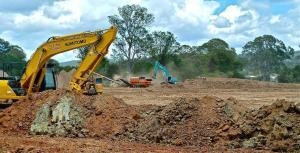## GleeYM

marketing@gleeym.com

# Earned Value Management (EVM) Concept

Earned Value (EV) has always been challenging to understand. EV covers 4 to 5 questions in the exam. I am going to narrate an example to explain this concept:

Q – A company ABC is hired to install underground pipes for water supply to metropolitan city. Total length is 9 km. The cost is \$ 30/Ft. Project duration is 6 months. Today 2nd month is completed. The Company has installed pipes over 1.8 km. The Cost incurred is \$ 185,000.

Calculate CV, CPI, SV, and SPI?

A – First need to calculate EV. EV is Budgeted cost multiplied by % complete i.e.

EV = Budgeted Cost x % Complete

The Cost to install one running foot of pipe is \$ 30. The Total distance is 9 km. The Cost is expressed per running feet and distance is taken in Kilometer. So unit conversion is required. Convert cost and distance into the same unit. Therefore Budgeted Cost is

Budgeted Cost = Total Cost (Some of PV)

= \$ 30/ft *9 km

= \$ 98.425/m * 9000 m

= \$ 885,825

Budgeted Cost (BC) = \$ 885,825

1.8 km is complete so far. It means

% complete = 1.8/9

= 0.2

= 20 %

% complete = 20 %

EV = 885,825 x .20

Ev = 177,165

Now Calculate

1 – Cost variance (CV):

CV = EV-AC

= 177,165 – 185,000

= – 7,835

CV = – 7,835

2 – Cost Performance Index (CPI):

CPI = EV/AC

= 0.95

CPI = 0.95

3 – Schedule Variance:

SV = EV – PV (Planned Value)

= 177,165 – 295,275

= – 118, 120

SV = 118,120

4 – Schedule Performance Index (SPI):

SPI = EV/PV

= 177,165/295,275 = 0.6

Project is cost overrun and behind schedule

* One ft is 304.8 mm, one m to1000 mm.

**PV is calculated as:

## More To ExploreUncategorized

### Real Estate Development Project – Better way to Envisage Your Project’s Future. How? 4 Graphs are Enough to Illustrate!

Keywords Real Estate, Real Estate Development Project, Cost Estimates, Schedule, Construction, Skilled Labor, Construction Equipment, Site Development, House Construction, Bricks, Facilities Development, Landscape, occupational HSE,Cost Risk Analysis

### Are Caring about the Penalty Clause in a Mega Construction Project? 3 Questions Need to be Addressed!

Key Words Construction, penalty, Delay, Contractor, Client, Owner, Construction Project, Project Delivery, Project Controls, Brown Field, Green Field, Cost Estimates, Schedule, Integrated Cost Risk Analysis,